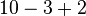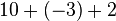# Order of Operations

When there is more than one operation involved in a mathematical problem, it must be solved by using the correct order of operations. Remember, calculators will perform operations in the order which you enter them, therefore, you will need to enter the operations in the correct order for the calculator to give you the right answer.

Rules

1. Calculations must be done from left to right.
2. Calculations in brackets (parenthesis) are done first. When you have more than one set of brackets, do the inner brackets first.
3. Exponents (or radicals) must be done next.
4. Multiply and divide in the order the operations occur.
5. Add and subtract in the order the operations occur.

 Remember to: Simplify inside groupings of parentheses, brackets and braces first. Work with the innermost pair, moving outward. Simplify the exponents. Do the multiplication and division in order from left to right. Do the addition and subtraction in order from left to right.

PEMDAS or Please Excuse My Dear Aunt Sally (Parenthesis, Exponents, Multiply, Divide, Add, Subtract)

BEDMAS (Brackets, Exponents, Divide, Multiply, Add, Subtract)

Big Elephants Destroy Mice And Snails (Brackets, Exponents, Divide, Multiply, Add, Subtract)

Examples

 12 ÷ 4 + 3212 ÷ 4 + 9 3 + 9 12 Rule 3: Exponent first Rule 4: Multiply or Divide as they appear Rule 5: Add or Subtract as they appear (42 + 5) – 3 21 – 3 18 Rule 2: Everything in the brackets first Rule 5: Add or Subtract as they appear 20 ÷ (12 – 2) X 32 – 2 20 ÷ 10 X 32 – 2 20 ÷ 10 X 9 – 2 18 – 2 16 Rule 2: Everything in the brackets first Rule 3: Exponents Rule 4: Multiply and Divide as they appear Rule 5: Add or Subtract as they appear

Mathematicians were very careful when they developed the order of operations.
Without the correct order, watch what happens:

15 + 5 X 10 — Without following the correct order, I know that 15+5=20 multiplied by 10 gives me the answer of 200.

15 + 5 X 10 — Following the order of operations, I know that 5X10 = 50 plus 15 = 65. This is the correct answer, the above is not!

You can see that it is absolutely critical to follow the order of operations. Some of the most frequent errors students make occur when they do not follow the order of operations when solving mathematical problems. Students can often be fluent in computational work yet do not follow procedures. Use the handy acronyms to ensure that you never make this mistake again.

According to Wikipedia:

Mnemonics are often used to help students remember the rules, but the rules taught by the use of acronyms can be misleading. In the United States the acronym PEMDAS is common. It stands for Parentheses, Exponents, Multiplication, Division, Addition, Subtraction. PEMDAS is often expanded to “Please excuse my dear Aunt Sally” with the first letter of each word creating the acronym PEMDAS. Canada uses BEDMAS and the UK uses BODMAS. In Canada and other English speaking countries, Parentheses may be called Brackets, or symbols of inclusion and Exponentiation may be called either Indices, Powers or Orders, and since multiplication and division are of equal precedence, M and D are often interchanged, leading to such acronyms as BEDMAS, BIDMAS, BODMAS, BERDMAS, PERDMAS, and BPODMAS.
These mnemonics may be misleading when written this way, especially if the user is not aware that multiplication and division are of equal precedence, as are addition and subtraction. Using any of the above rules in the order “addition first, subtraction afterward” would also give the wrong answer.The correct answer is 9 (and not 5, which we get when we add 3 and 2 first to get 5,and then subtract it from 10 to get the final answer of 5), which is best understood by thinking of the problem as the sum of positive ten, negative three, and positive two.An alternative way to write the mnemonic is:

P

E

MD

AS

Or, simply as PEMA, where it is taught that multiplication and division inherently share the same precedence; and that addition and subtraction inherently share the same precedence. PEMA is one of the mnemonics taught in New Zealand.

This makes the equivalence of multiplication and division, and addition and subtraction, clear.

Another potentially misleading aspect of this mnemonic is the inclusion of P for parentheses. First of all, parentheses are grouping symbols, not operation symbols. Also, within the grouping symbols, there may be expressions involving several operations, which would need to be evaluated according to the correct order or operations which appear AFTER the P in the mnemonic. It is probably better to teach that grouping symbols are not operations themselves, but rather are used to change the precedence of operations from the default.

Once logs are introduced they should be given the same precedence as exponents.